# Calculus 1 : Relative Maximum

## Example Questions

### Example Question #103 : Intervals

Given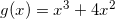Find the-coordinate of the relative maximum on the interval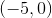.

Cannot be determined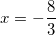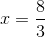Explanation:

To find relative maximums, we need to find where our first derivative changes sign. To do this, find your first derivative and then find where it is equal to zero.

Begin with: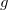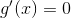at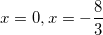This means we have extrema at x=0 and x=-8/3

Because we are only concerned about the interval from -5 to 0, we only need to test points on that interval. Try points between our two extrema, as well as one between -8/3 and -5.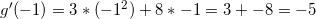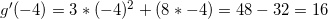So our first derivative goes from negative to positive at -8/3, thus this is the x coordinate of our relative maximum on this interval.

### Example Question #1 : How To Find Relative Maximum On The Interval By Graphing Functions

Where is the maximum value of the functionon the interval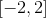?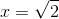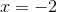Explanation:

Though there are relative maxima at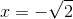and(found by setting the first derivative of the function equal to zero and solving for x.)  the maximum value along the whole interval is actually at the upper endpoint, when.  When looking for extrema along an interval, looking for zeros of the first derivative does not account for endpoint extrema.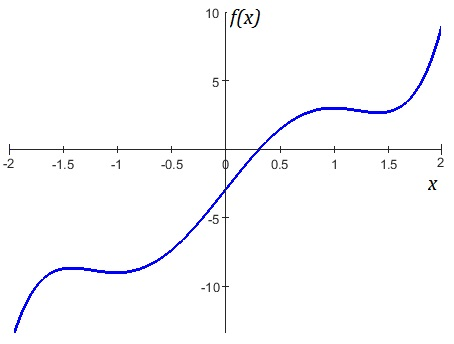### Example Question #105 : Intervals

Determine the relative maxima for the function: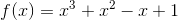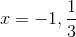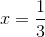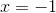Explanation:

To determine the relative maxima for the function, we must determine where the first derivative of the function changes from positive to negative.

The first derivative of the function is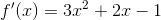and was found using the following rule: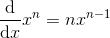Next, we must find the critical points, the values at which the first derivative is equal to zero: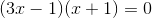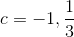Using the critical values, we can then create the intervals on which we evaluate the sign of the first derivative: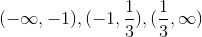To check the sign of the first derivative, plug in any value on each interval into the first derivative function. On the first interval, the first derivative is positive, on the second, it is negative, and on the third it is positive. The first derivative changes from positive to negative atso a relative maximum exists here.

### Example Question #106 : Intervals

Find the local max of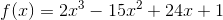using the equation and/or the following graph.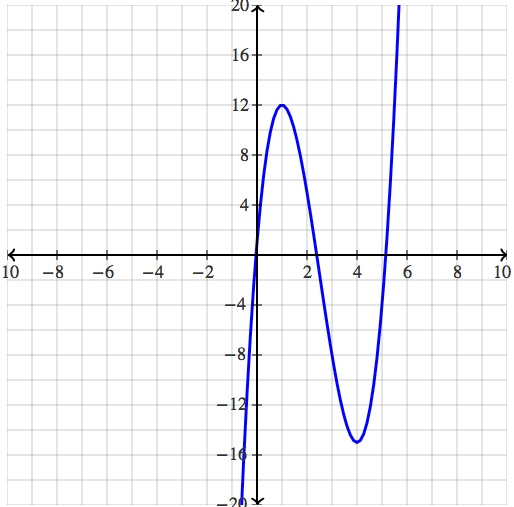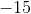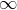Explanation:

We notice that this function has 2 extrema which are located atand. We could have also of found this by looking at the function itself:.

We know that extrema exist when the slope of the function is zero, hence we take the derivative, set it equal to zero, and than solve for x.

The derivative is the following: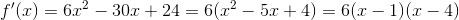.

Therefore setting both pieces equal to zero we see the following: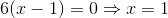and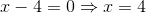.

Finding out if one of these x values produce a local min or max however requires either the first derivative test, the second derivative test, or analyzing the graph. We see that for a small neighborhood around,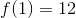is the largest term, hence it is a local max.  Similarly, for a small neighborhood around,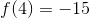is the smallest term, hence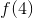is a local min.

Therefore whenis the only spot in which a local max occurs. Remember that it is not the x value that must be the largest, rather it is it's corresponding y value.

### All Calculus 1 Resources